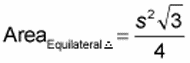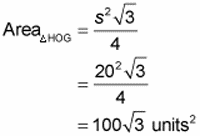##### Geometry For DummiesTo calculate a triangle’s area — for most types of triangles — you need to know the triangle’s base and height. However, with an equilateral triangle, all you need to know is the length of one of its sides. You can then plug it into the following formula to quickly calculate the area.

Area of an equilateral triangle (with side s):Here’s the formula in action:

What’s the area of a triangle HOG whose sides measure 20 units?